# Axway Integration Builder – Variable PersistenceFlow Value variables are defined in the flow and set in the instance. Each time the flow executes the value is reset to the value in the instance. What if you’d like to modify the variable and have the value persist between flow executions? Why would I even want to do this?

One reason you may want to have a variable persist between flows is if the flow needs to query a data source to see what changed since the last execution. In this case you will need to store the time stamp of the last execution. While you could store the variable in an external database, with Axway’s Integration Builder there is no need to do this. This blog post will describe how to use Platform API requests to store a value variable between flow executions.

Here are the basic steps:

• Create a flow value variable, e.g. lastExecutionTime
• Read the value variable using config.lastExecutionTime
• Update the variable as follows:
• Retrieve the formula instance metadata using the GET /formulas/{id}/instances/{instanceId} platform API
• Modify the variable with the value you’d like to store
• Update the formula instance using the PUT /formulas/{id}/instances/{instanceId} platform API

Let’s dive in.

## Create a Value Variable

• Create a new flow and select scheduled triggered for the type. I hard coded the Cron value for the schedule to once per minute ( 0 * * ? * * )
• Create a new Value variable called lastExecutionTime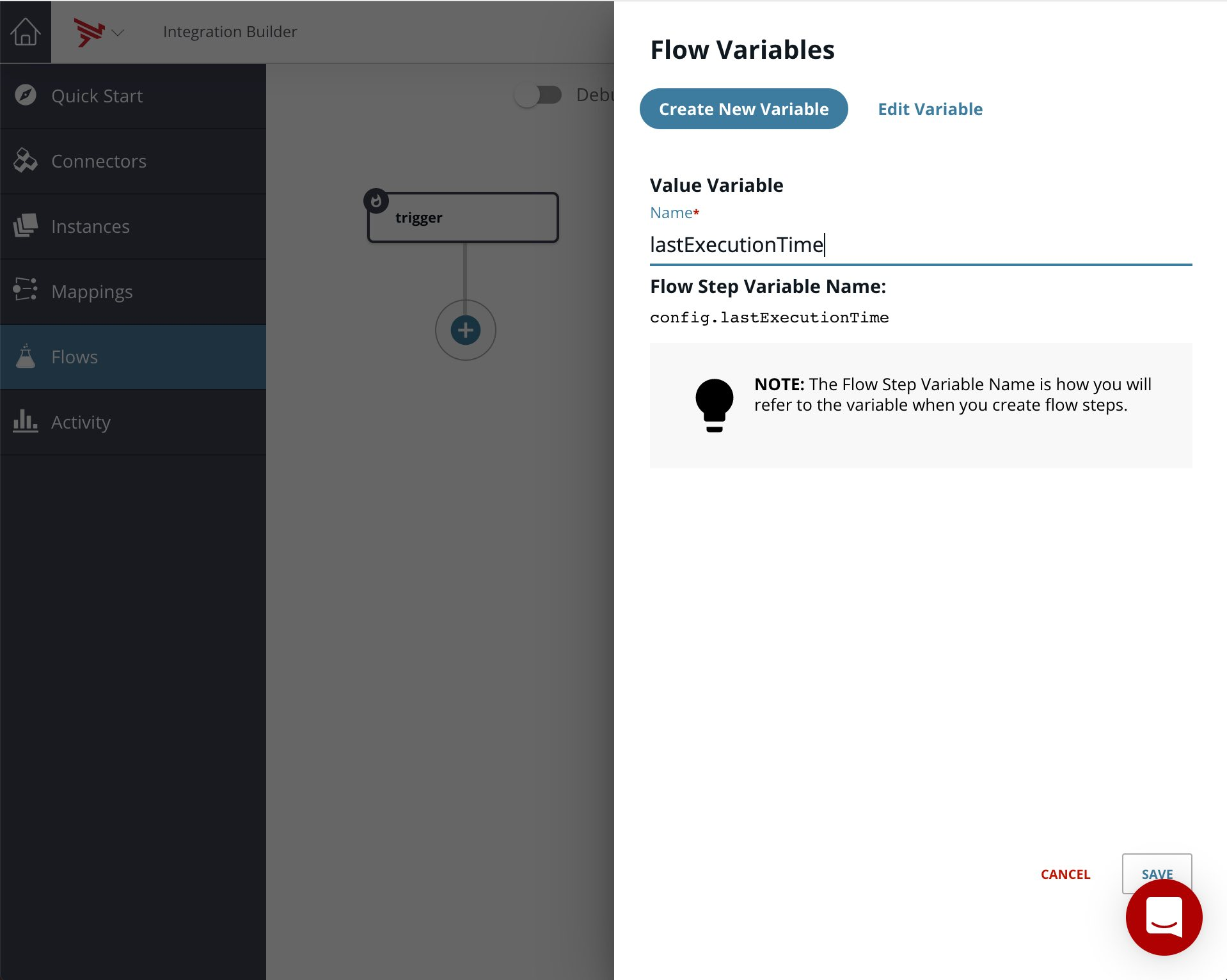• Add a JS Step to print the variable to the console log. My step is called consoleLog.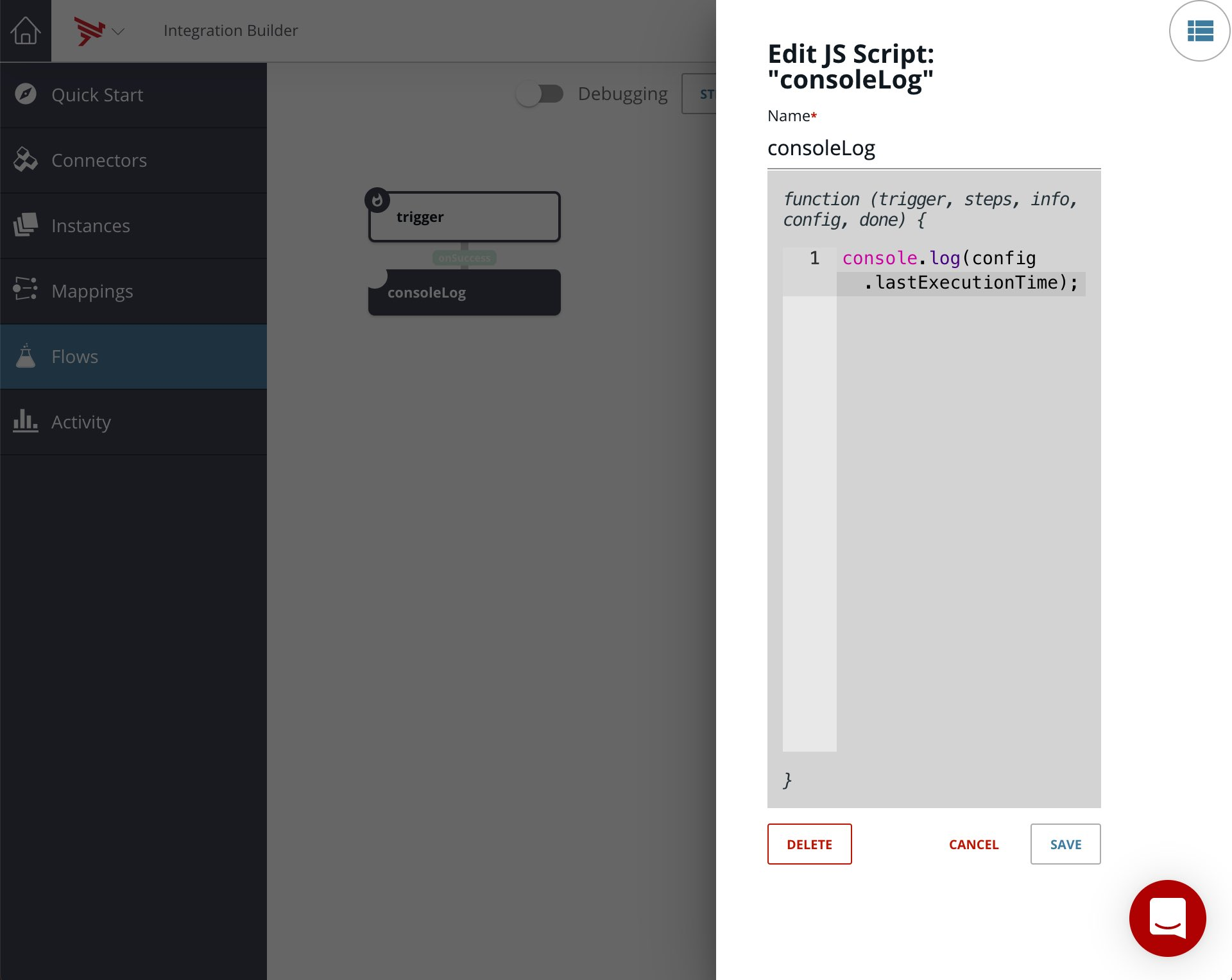## Update the Variable

### Retrieve the Formula Instance Metadata

• Create a JS Step to create the url to the platform API GET /formulas/{id}/instances/{instanceId} as follows: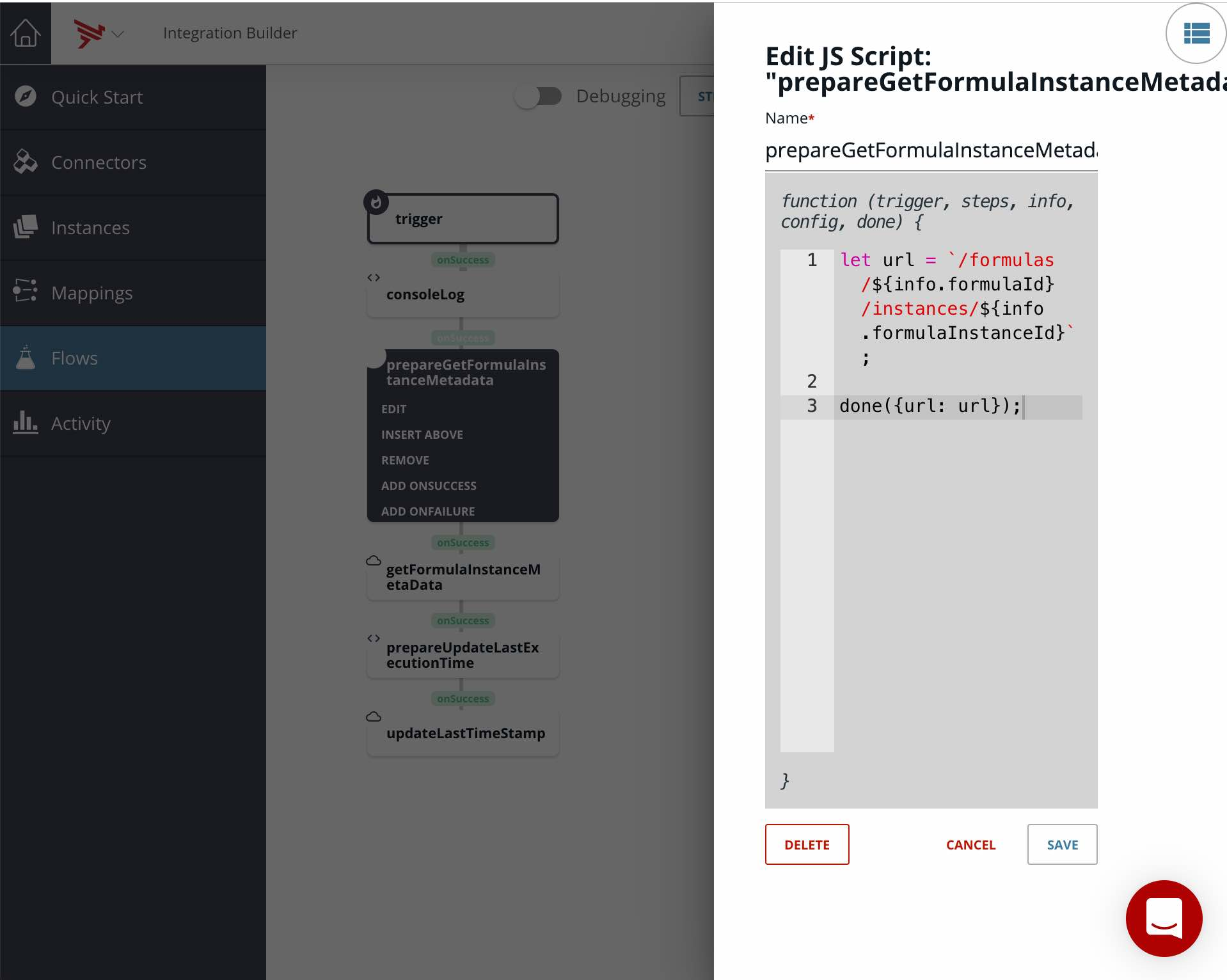``````let url = `/formulas/\${info.formulaId}/instances/\${info.formulaInstanceId}`;

done({url: url});
``````

Note that my step is called prepareGetFormulaInstanceMetadata.

• Make the Platform API Request as follows: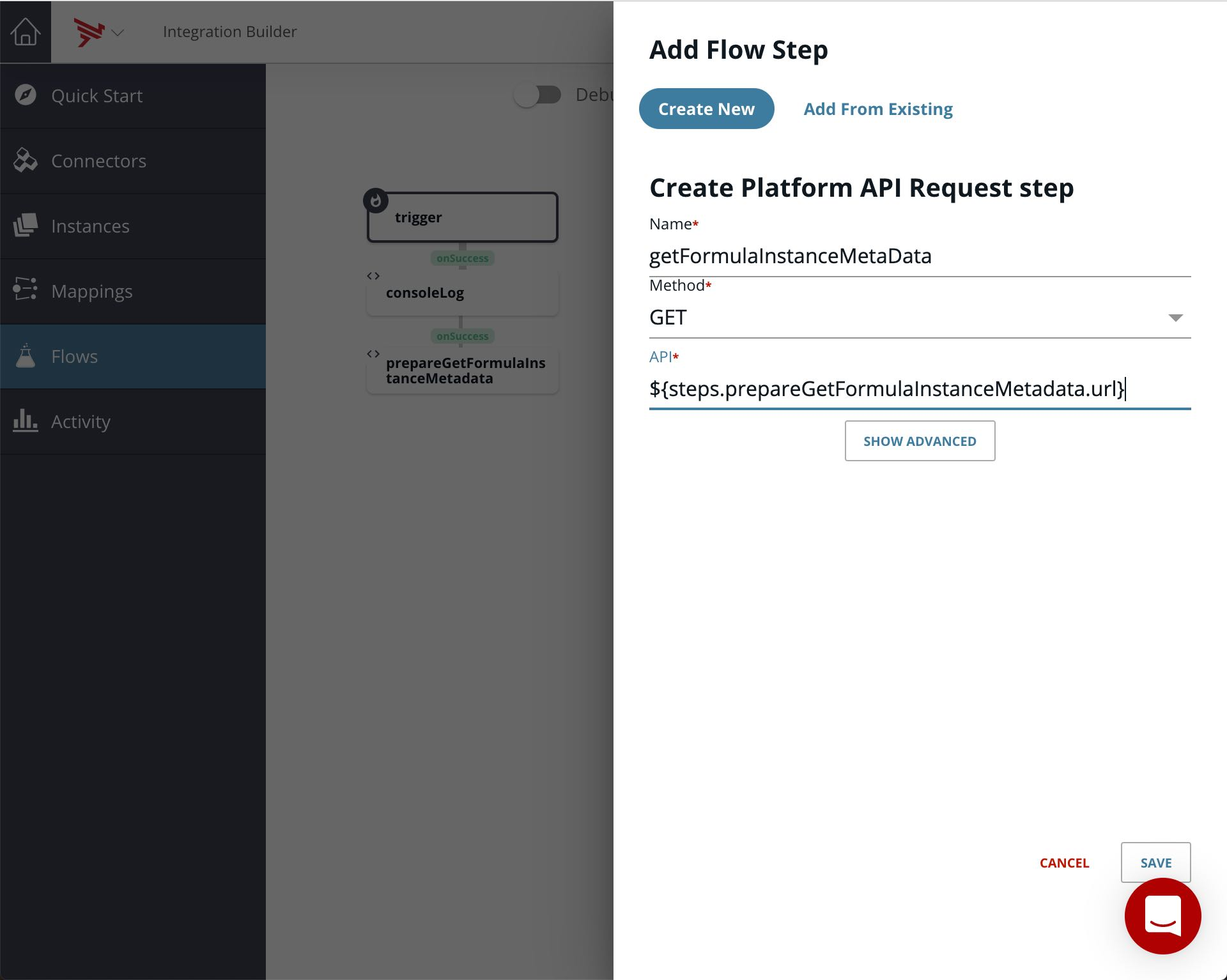Note that my step is called getFormulaInstanceMetaData.

### Modify the Value Variable

We will do this in the same step as updating the formula instance below.

### Update the Formula Instance

Now we can update the formula instance with our revised variable value so that it can be accessed during the next execution of the flow.

• Create a JS Step to update the value variable (in the formula instance)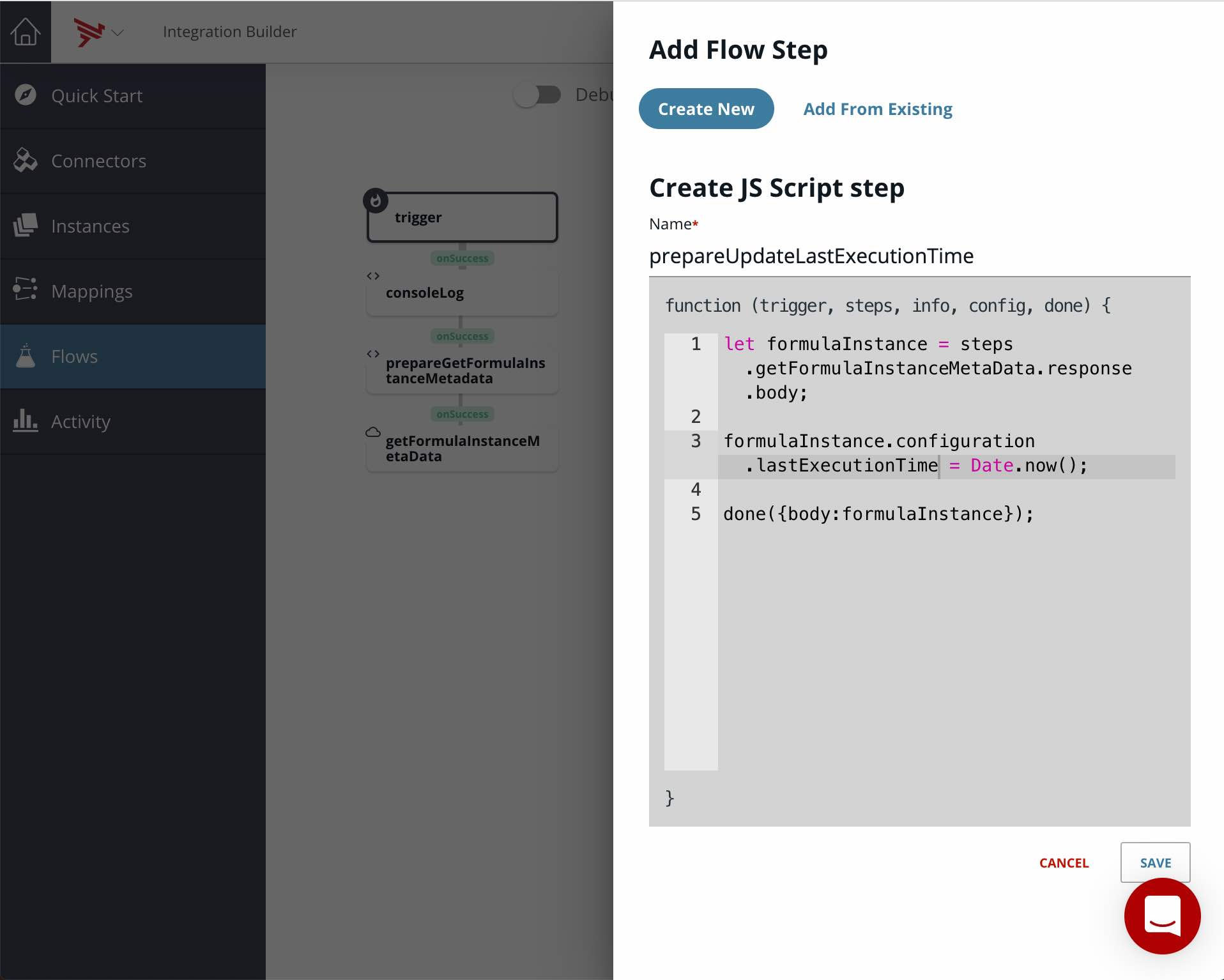``````let formulaInstance = steps.getFormulaInstanceMetaData.response.body;

formulaInstance.configuration.lastExecutionTime = Date.now();

done({body:formulaInstance});
``````

Note that we are setting the value variable lastExecutionTime to the current time.

Note that my step is called prepareUpdateLastExecutionTime.

• Make the Platform API Request as follows: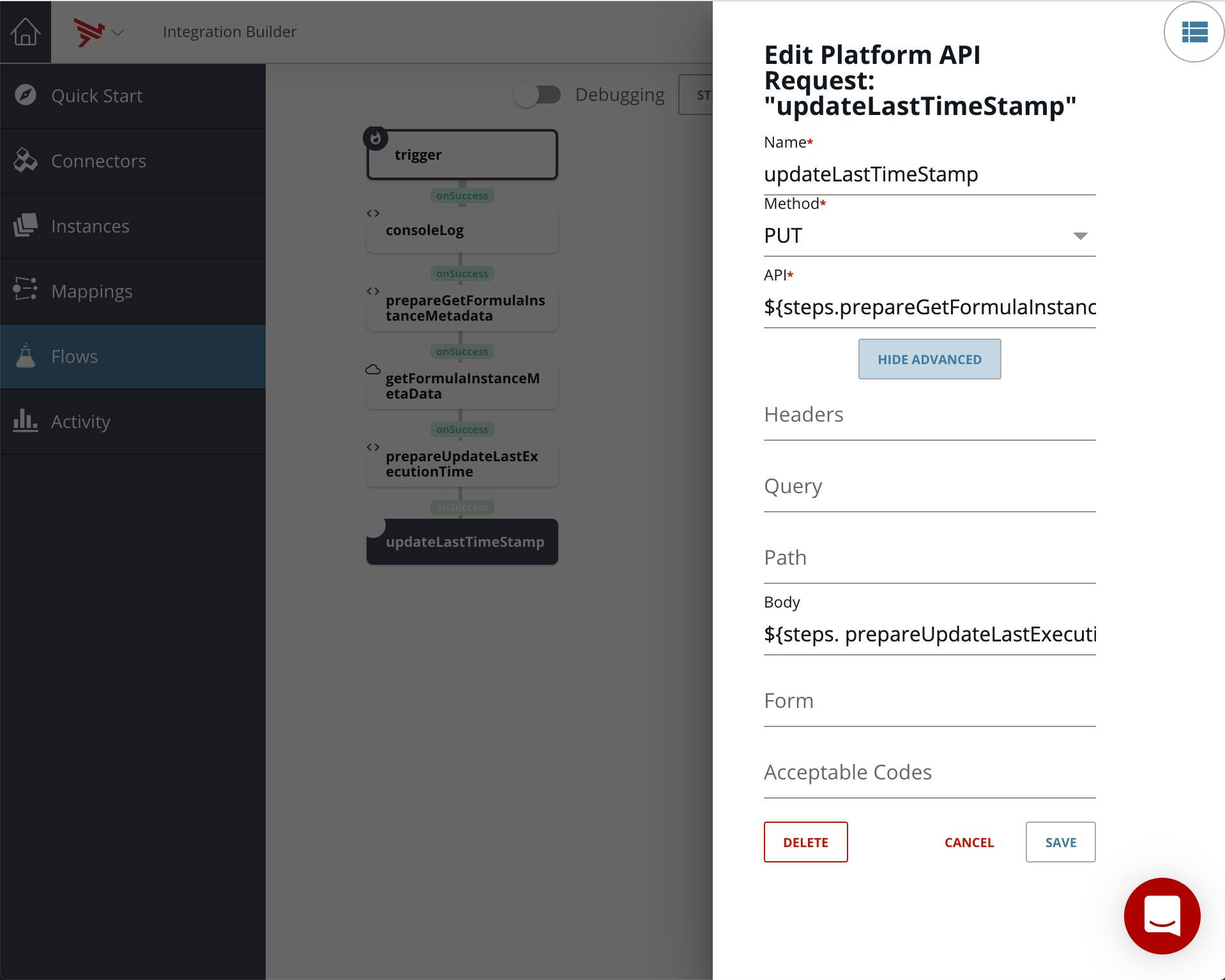Note that we are using the url calculated earlier since it is the same

Note that my step is called updateLastTimeStamp.

The entire flow should look as follows: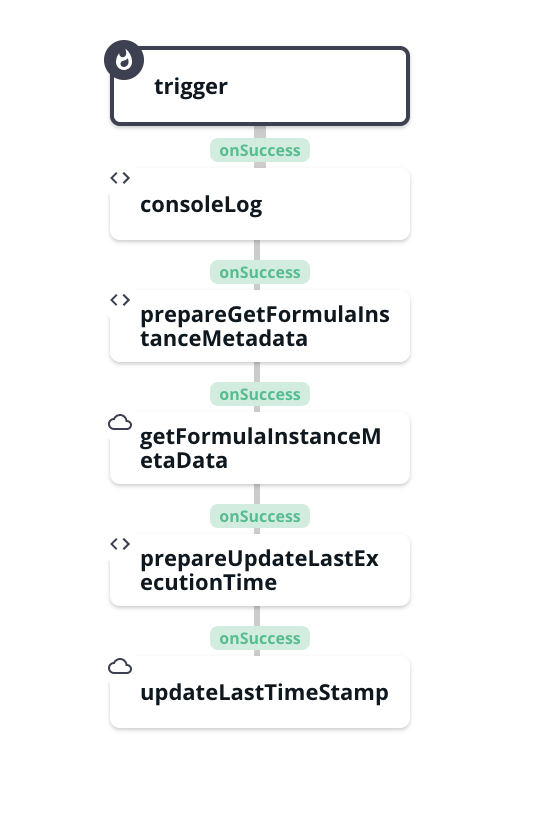## Test Flow

Let’s test our flow by creating an instance setting lastExecutionTime to 0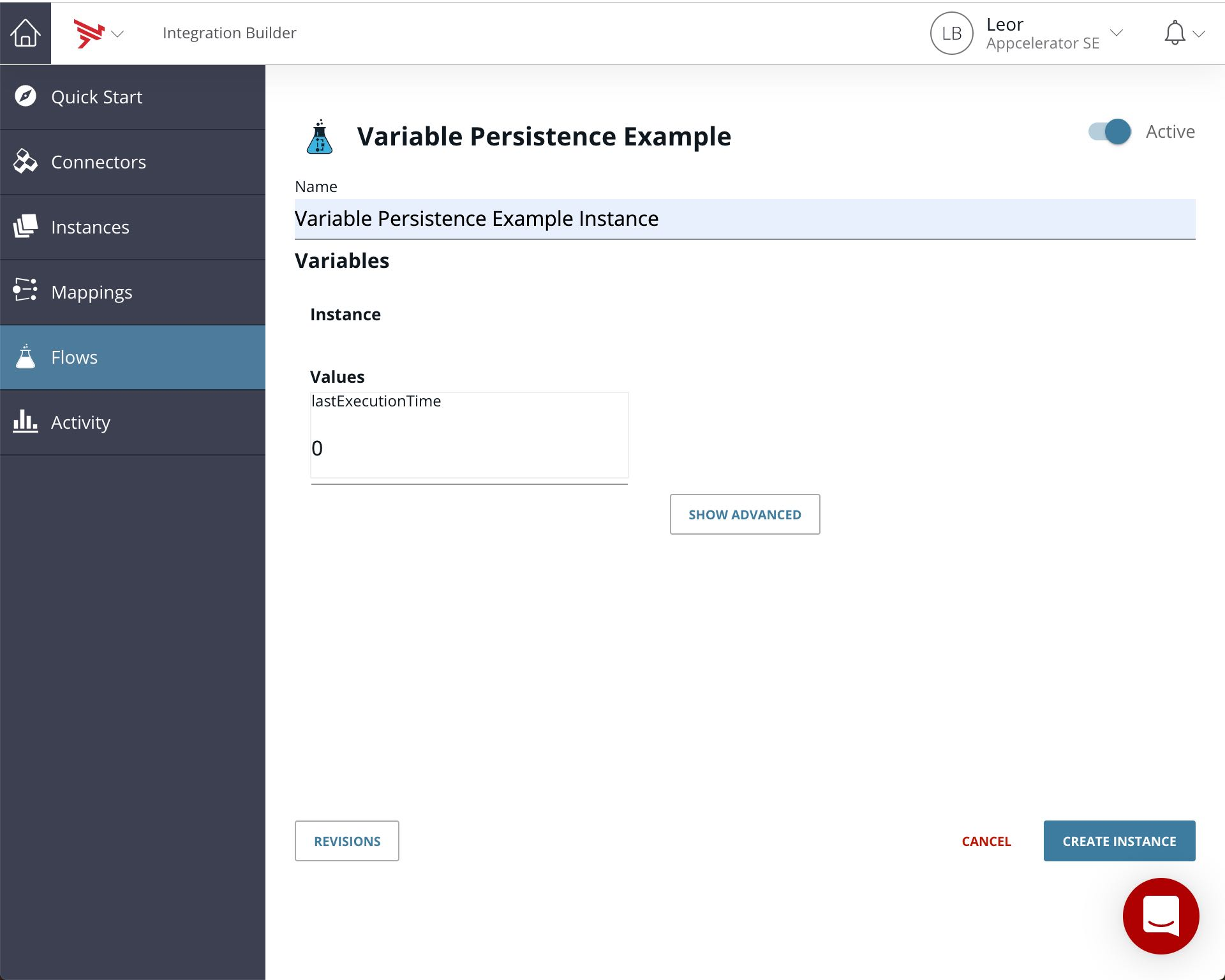After a few minutes you should see the flow has executed a few times as follows: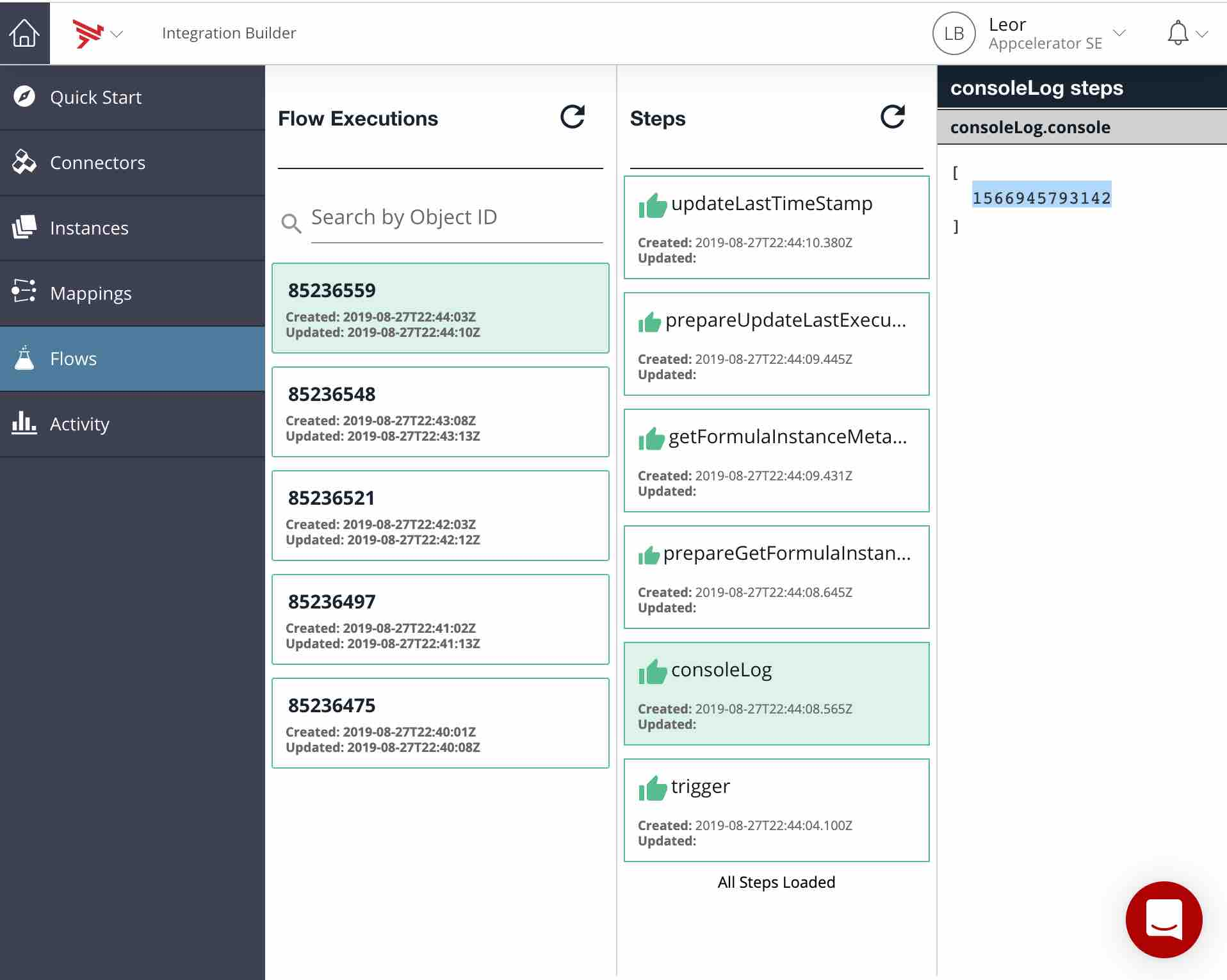If you look at the consoleLog step you will see time stamps similar to below:

• 0
• 1566945607780
• 1566945670948
• 1566945731649
• 1566945793142

The first 0 is the variable value that was set in the instance. The remaining are time stamps for each flow execution.

You can check this web site to convert the timestamps to a human readable time and you will see that there is roughly 1 minute between each timestamp. This is the cron interval we set when creating the flow.

## Summary

In this blog post we saw how it is possible to persist data between flow executions, which would be useful for applications that need to query data that has changed since last check as well as other use cases.

You can find my test flow here.